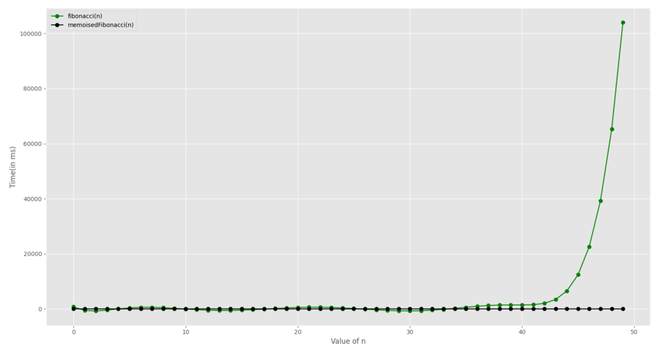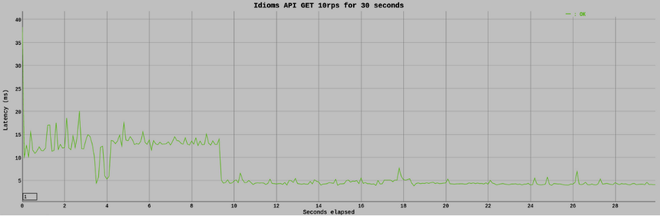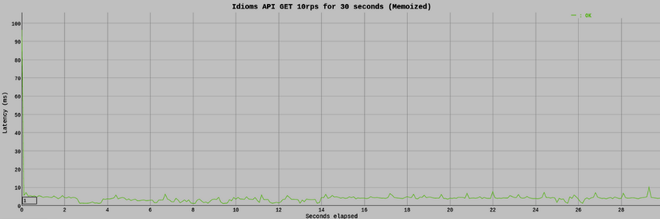GFG App
Open AppBrowser
Continue

As our systems mature and begin to do more complex calculations, the need for speed grows, and process optimization becomes a need. When we overlook this issue, we end up with applications that take a long time to run and demand a large number of system resources.

In this article, we are going to look at memoization which is one technique that can help us significantly reduce processing time if done the right way.

Memoization: Memoization is a technique for speeding up applications by caching the results of expensive function calls and returning them when the same inputs are used again.

Let us try to understand this by breaking the definition into small parts.

• Expensive Function Calls: Time and memory are the two most important resources in computer applications. As a result, an expensive function call is one that consumes large amounts of these two resources due to extensive calculation during execution.
• Cache: A cache is just a temporary data store that stores data in order to serve future requests for that data more quickly.

Importance of Memoization: When a function is given in input, it performs the necessary computation and saves the result in a cache before returning the value. If the same input is received again in the future, it will not be necessary to repeat the process. It would simply return the cached answer from the memory. This will result in a large reduction in a code’s execution time.

Memoization in Javascript: In JavaScript, the concept of memorization is based mostly on two ideas. They are as follows:

• Closures
• Higher-Order Functions

Closures: Before talking about closure, let’s take a quick look at the concept of lexical scope in JavaScript. Lexical scoping defines the scope of a variable by the position of that variable declared in the source code.

Example:

## Javascript

 `let hello = ``"Hello"``; ` ` `  `function` `salutation() {  ` `    ``let name = ``"Aayush"``;  ` `    ``console.log(`\${hello} \${name}!`);  ` `}`

In the above code:

• The variable hello is a global variable. It can be accessed from any location, including the salutation() function.
• The variable name is a local variable that can only be accessed within the salutation() function.

According to lexical scoping, the scopes can be nested and the inner function can access the variables declared in its outer scope. Hence in the code below, the inner function greet() has access to the variable name.

## Javascript

 `function` `salutation() {  ` `    ``let name = ``"Aayush"``; ` `    ``function` `greet() { ` `        ``console.log(`Hello \${name}!`); ` `    ``} ` `    ``greet();  ` `}`

Now let’s modify this salutation() function and rather than invoking the function greet(), we return the greet() function object.

## Javascript

 `function` `salutation() { ` `    ``let name = ``'Aayush'``; ` ` `  `    ``function` `greet() { ` `        ``console.log(`Hello \${name}!`); ` `    ``} ` `    ``return` `greet; ` `} ` ` `  `let wish = salutation(); ` `wish();`

If we run this code, we will get the same output as before. However, it’s worth noting that a local variable is generally only present during the function’s execution. It means that when salutation() execution is completed, the name variable is no longer accessible. In this case, when we execute wish(), the reference to greet(), the name variable still exists. A closure is a function that preserves the outer scope in its inner scope.

Higher-Order Functions: Higher-order functions are functions that operate on other functions by taking them as arguments or returning them. For example, in the above code, salutation() is a higher-order function.

Now, using the famous Fibonacci sequence, let’s examine how memoization makes use of these concepts.

Fibonacci Sequence: The Fibonacci sequence is a series of numbers that begins with one and ends with one, following the rule that each number (called a Fibonacci number) is equal to the sum of the two numbers before it.

`1, 1, 2, 3, 5, 8, 13, 21, 34, 55, ...`

A simple recursive solution to this problem would be:

## Javascript

 `function` `fibonacci(n) { ` `    ``if` `(n < 2) ` `        ``return` `1; ` `    ``return` `fibonacci(n - 1) + fibonacci(n - 2); ` `}`

If we were to plot the recursion tree for the above function when n=4, it would look like this,As you may notice, there are too many redundant computations.

Let’s try to fix this with memoization.

## Javascript

 `function` `memoisedFibonacci(n, cache) { ` `    ``cache = cache || [1, 1] ` `    ``if` `(cache[n]) ` `        ``return` `cache[n] ` `    ``return` `cache[n] = memoisedFibonacci(n - 1, cache) +  ` `    ``memoisedFibonacci(n - 2, cache); ` `}`

We change the function in the code sample above to accept an optional argument called cache. We use the cache object as a temporary memory to store Fibonacci numbers with their associated indices as keys, which can then be retrieved as needed later in the execution.If we plot the execution time, for both versions of the Fibonacci function, it is quite evident that the employment of the memoization technique leads to significant time reduction.

Practical Example: Javascript Memoization for a Web Response: To demonstrate this, we’ll use an example Idioms API. It is a simple REST API built using Node.js.

Response times before Memoization: Following is a simple Express.js route that returns all of the idioms stored within the API. In this situation, each call will result in a database query.

## Javascript

 `import express from ``'express'``; ` `const router = express.Router(); ` `import { getAllIdioms } from ``'../services/database.js'``; ` ` `  `router.get(``'/'``, async ``function``(req, res, next) { ` `    ``try` `{ ` `        ``res.json(await getAllIdioms()); ` `    ``} ``catch` `(err) { ` `        ``console.log(``'Error while getting idioms '``, err.message); ` `        ``res.status(err.statusCode || 500).json({ ` `            ``'message'``: err.message ` `        ``}); ` `    ``} ` `})`

Let’s have a look at how long it takes for this approach to respond. I ran a quick load test using the Vegeta load testing tool.Response times after Memoization: Now let’s modify the above code to add memoization. For the purposes of this illustration, I’ve used the p-memoize package.

## Javascript

 `import express from ``'express'``; ` `const router = express.Router(); ` `import { getAllIdioms } from ``'../services/database.js'``; ` `import pMemoize from ``'p-memoize'``; ` `const ONE_MINUTE_IN_MS = 60000; ` `const memGetAllIdioms = pMemoize(getAllIdioms, { maxAge: ONE_MINUTE_IN_MS }); ` ` `  `router.get(``'/'``, async ``function` `(req, res, next) { ` `    ``try` `{ ` `        ``res.json(await memGetAllIdioms()); ` `    ``} ``catch` `(err) { ` `        ``console.log(``'Error while getting idioms '``, err.message); ` `        ``res.status(err.statusCode || 500).json({``'message'``: err.message}); ` `    ``} ` `})`

As a result,When compared to the previous graph, we can observe that the Express.js route that uses memoization is significantly faster than the non-memoize equivalent.

My Personal Notes arrow_drop_up